Next: Electric Field of a Up: Gauss' Law Previous: Electric Field of a

## Electric Field of a Uniformly Charged Wire

Consider a long straight wire which carries the uniform charge per unit length. We expect the electric field generated by such a charge distribution to possess cylindrical symmetry. We also expect the field to point radially (in a cylindrical sense) away from the wire (assuming that the wire is positively charged).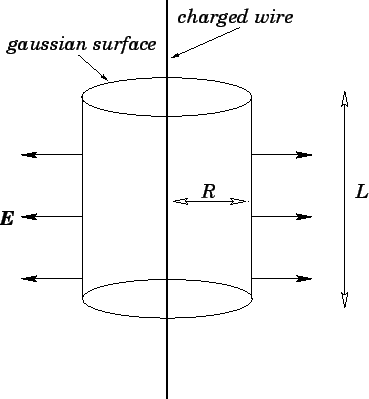Let us draw a cylindrical gaussian surface, co-axial with the wire, of radiusand length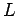--see Fig. 11. The above symmetry arguments imply that the electric field generated by the wire is everywhere perpendicular to the curved surface of the cylinder. Thus, according to Gauss' law,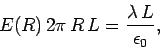(70)

where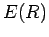is the electric field-strength a perpendicular distancefrom the wire. Here, the left-hand side represents the electric flux through the gaussian surface. Note that there is no contribution from the two flat ends of the cylinder, since the field is parallel to the surface there. The right-hand side represents the total charge enclosed by the cylinder, divided by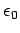. It follows that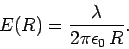(71)

The field points radially (in a cylindrical sense) away from the wire if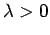, and radially towards the wire if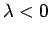.Next: Electric Field of a Up: Gauss' Law Previous: Electric Field of a
Richard Fitzpatrick 2007-07-14